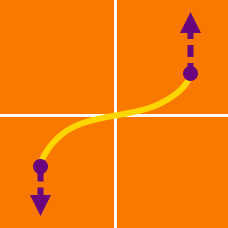Calculus

# Arc Length by Integration

If $${f}'(x) = \sqrt{ {x}^4-1 },$$ what is the length of the curve $$y = f(x)$$ from $$x = 2$$ to $$x = 8?$$

What is the length of the curve $$y = \frac{1}{2}( {e}^x+{e}^{-x} )$$ from $$x = -13$$ to $$x = 13$$?

Let $$L$$ be the length of the line $$y = 3x + 4$$ on the interval $$3 \leq x \leq 8$$. If $$L = \sqrt{a}$$, then what is the value of $$a$$?

Given $$y \geq 0 ,$$ what is the length of the curve $${y}^2 = {x}^3$$ on the interval $$0 \leq x \leq 4$$?

Let $$L$$ be the length of the curve $$\displaystyle{y = \frac{1}{4}{x}^2 - \frac{1}{2} \ln x }$$ for $$4 \leq x \leq 8.$$ If $L = a+\frac{1}{2}\ln{b} ,$ where $$a$$ and $$b$$ are positive integers, what is the value of $$a+b?$$

×